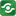NPR Permutations Calculator@import url(http://www.google.com/cse/api/branding.css);CSGNetwork Custom SearchCSGNetwork Snippets Calculators Top Free Apps Home Back

Right click this window and select "view source" in order to copy the source for this script.

 Value Of n Value Of r Permutations(n,r)

This calculation is the number of ways of obtaining an ordered subset of r elements from a set of n elements. n = the set size. It is the total number of items in the sample. r = the subset size. It is the number of items chosen from the sample. Only whole positive (integer) numbers are valid. Permutations gives the number of ways a subset of r items can be chosen out of a set of n items and different arrangements of the same items are also counted. For r <= n, n >= 0, and r >= 0. The permutations formula is (P(n,r) = n! / (n - r)!)...

NPR Permutations Calculator

This will calculate the permutations for P(n,r) = n! / (n - r)!.

View the source...